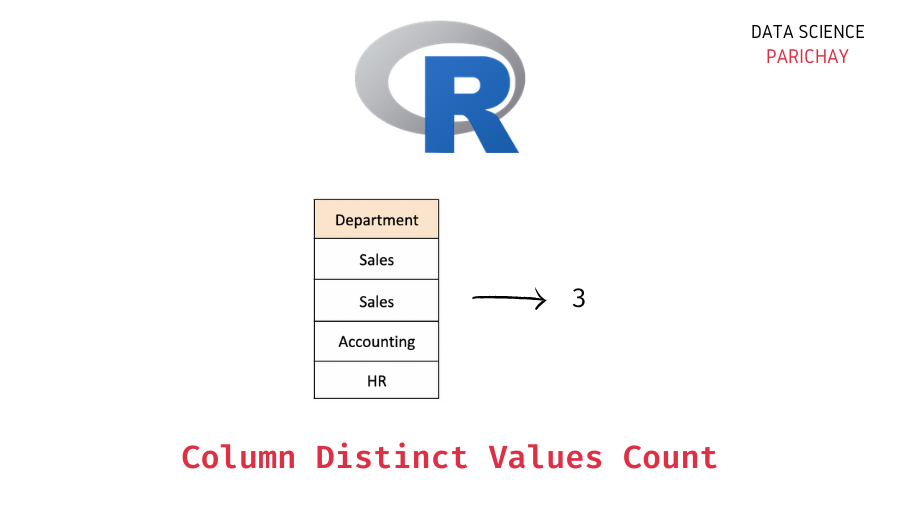# R – Count Unique Values in Dataframe Column

A dataframe in R is a data structure used to store tabular data in rows and columns. In this tutorial, we will look at how to count the unique values in a column of an R dataframe with the help of some examples.

## How do you get the unique values count of an R dataframe column?

You can use a combination of the `length()` function and the `unique()` function in R to get the count of unique values in a dataframe column.

The idea is to first get the unique values from the column in a vector using the `unique()` function and then apply `length()` function on this unique values vector to get a count of the unique values in the column.

The following is the syntax –

`length(unique(dataframe[[columan_name]]))`

We get the unique values count in the column as an integer.

## Steps to count distinct values in an R dataframe column

Let’s now look at the steps to follow to get distinct values in a dataframe column in R

### Step 1 – Create a dataframe

First, we will create a dataframe that we will be using throughout this tutorial.

```# create a dataframe
employees_df = data.frame(
"Name"= c("Jim", "Dwight", "Angela", "Tobi", "Kevin"),
"Age"= c(26, 28, 29, 32, 30),
"Department"= c("Sales", "Sales", "Accounting", "HR", "Accounting")
)
# display the dataframe
print(employees_df)```

Output:

📚 Data Science Programs By Skill Level

Introductory

Intermediate ⭐⭐⭐

🔎 Find Data Science Programs 👨‍💻 111,889 already enrolled

Disclaimer: Data Science Parichay is reader supported. When you purchase a course through a link on this site, we may earn a small commission at no additional cost to you. Earned commissions help support this website and its team of writers.

```    Name Age Department
1    Jim  26      Sales
2 Dwight  28      Sales
3 Angela  29 Accounting
4   Tobi  32         HR
5  Kevin  30 Accounting```

We now have a dataframe containing information about some employees working in an office. The dataframe has columns “Name”, “Age”, and “Department”.

### Step 2 – Get the count of the unique values in the column using `unique()` and `length()`

To get the unique value count in a column, pass the column values vector to the `unique()` function as an argument. You can use the `[[]]` notation to get a column’s values vector. This will result in a vector of unique values from the column.

Then, you can then apply the `length()` function on this vector of unique values to get the unique values count.

Let’s get the count of distinct departments from the “Department” column in the above dataframe.

```# get count of distinct values in "Department" column
dept_count <- length(unique(employees_df[["Department"]]))
# display the result
print(dept_count)```

Output:

` 3`

We get the number of unique values in the “Department” column as 3.

Note that you can also use the column index to access a column’s values. For example, in the above dataframe, the index of the “Department” column is 3 (rows and columns in R are indexed starting from 1).

```# get count of distinct values in "Department" column
dept_count <- length(unique(employees_df[]))
# display the result
print(dept_count)```

Output:

` 3`

We get the same result as above.

## Summary

In this tutorial, we looked at how to count the unique values in the column in an R dataframe. The following is a short summary of the steps –

1. Create a dataframe (skip this step if you already have a dataframe on which you want to operate).
2. Use a combination of the `unique()` and the `length()` functions in R to count the unique values in a column.

You might also be interested in –

•